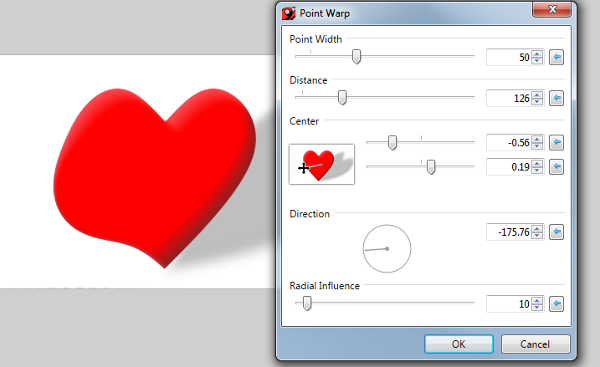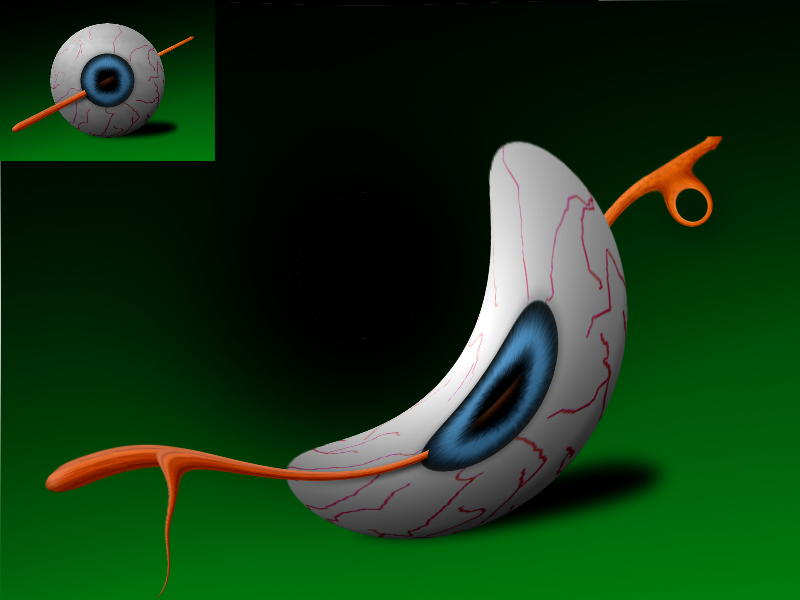## Recommended Posts

Point Warp

This is simply a rebuild of @Illnab1024's original plugin to make it compatible with paint.net v4. All credit goes to him. I've also reduced the source code from a Visual Studio project to a CodeLab script.

Effects -> Distort -> Point WarpFeatures

• Applies a 'point warp' at a given location on the image. It's basically bulge but in the horizontal plane instead of the vertical.

Changelog

v1.2 by toe_head2001 (Aug 20, 2015)

• Changed: Minor Changes to the source code to make it CodeLab compatible.

v1.1 by Illnab1024 (2008?)

• Small modifications to the UI and code that should look and work more intuitive.

v1.0 by Illnab1024 (Jan 10, 2008)

• Initial release

Source

Spoiler
```
// Name: Point Warp
// Author: Illnab1024
// Title:
// Version:
// Desc:
// Keywords:
// URL:
// Help:
#region UICode
int Amount1 = 10; // [0,150] Point Width
int Amount2 = 10; // [0,500] Distance
Pair<double, double> Amount3 = Pair.Create(0.0, 0.0); // Center
double Amount4 = 45; // [-180,180] Direction
int Amount5 = 10; // [0,200] Radial Influence
#endregion

void Render(Surface dst, Surface src, Rectangle rect)
{
double newRad = Amount5 * 1000;
Rectangle selection = EnvironmentParameters.GetSelection(src.Bounds).GetBoundsInt();
long CenterX = (long)(((selection.Right - selection.Left) / 2) + selection.Left);
long CenterY = (long)(((selection.Bottom - selection.Top) / 2) + selection.Top);
double offx, offy, trux, truy, xd, yd, Amnt, influence, fox, foy, angle;
float d;
Amnt = Math.Log(Math.Pow(System.Math.E * radius, Amount1)); // Better for getting an actual width of the cap.

angle = Amount4 * Math.PI / 180d;
angle += Math.PI;
offx = Math.Cos(angle) * Amount2;
offy = Math.Sin(angle) * Amount2;
d = (float)Amnt;
fox = (Amount3.First) * (rect.Width / 2);
foy = (Amount3.Second) * (rect.Width / 2) * -1;
for (int y = rect.Top; y < rect.Bottom; y++)
{
if (IsCancelRequested) return;
for (int x = rect.Left; x < rect.Right; x++)
{
xd = (double)(x - fox);
yd = (double)(y + foy);
influence = (System.Math.Pow((radius * System.Math.E), ((0d - (System.Math.Pow(((xd - CenterX) / (d)), 2) + System.Math.Pow(((yd - CenterY) / (d)), 2))))));
//influence = 0d - influence; --I honestly don't understand why I put that there 9 months ago.
trux = (offx * influence);
truy = (offy * influence);
trux += (double)x;
truy += (double)y;
ColorBgra pt = src.GetBilinearSample((float)(trux), (float)(truy));
// This now should not call out of the boundaries with the influence line gone,
// apart from amounts that create a great influence atop the cap of the function.

//pt.R = (byte)(((pt.A / 255) * (pt.R)) + ((1 - (pt.A / 255)) * (src[x, y].R)));
//pt.G = (byte)(((pt.A / 255) * (pt.G)) + ((1 - (pt.A / 255)) * (src[x, y].G)));
//pt.B = (byte)(((pt.A / 255) * (pt.) + ((1 - (pt.A / 255)) * (src[x, y].));
//pt.A = (byte)(255);
dst[x, y] = pt;
}
}
}```

•5

##### Share on other sites

Thanks toe-y! How about releasing this in it's own thread to differentiate the two versions?

Edit: New plugin split into it's own thread.

##### Share on other sitesEdited by Eli

##### Share on other sites

UI and effect screenshot.Feel free to add this into the first post toe-y.

•1

## Join the conversation

You can post now and register later. If you have an account, sign in now to post with your account.